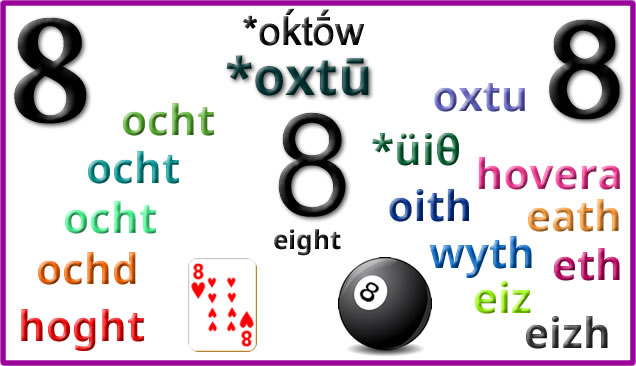# Eightsome

Words for eight and related things in Celtic languages:Proto-Celtic *oxtū = eight *oxtūmetos = eighth ocht [ˈoxt] = eight ochtmad [ˈoxtṽað] = eighth ochtar = a group of eight people ochtmoga = eighty ocht = eight ochtmad = eighth ochtur, ochtor, ochtar = eight people/things ochta = a group of eight things, an octad ochtmoga, ochtmogo, ochtmogat = eighty ocht [ɔxt̪ˠ/ʌxt̪ˠ]= eight ochtar = eight (people) ochtú = eighth, eighth part ocht déag = eighteen ochtó = eighty ochtddach = having eight parts, eightfold ochtábhó = octavo ochtach, ochtáibh = octave ochtagán = octagon ochtapas = octopus ochd [ɔxg] = eight ochdamh [ɔxgəv] (8ᵐʰ) = eighth (8ᵗʰ) ochdnar [ɔxgnər] = eight people ochd-fillte = octuple, eightfold, eight-ply ochd-shliosach = octagon, octahedron ochd-chasach = octopus hoght [hoːx(t)] = eight, octuple hoghtoo = eighth hoght jeig = eighteen hoghtad = eighty hoght filley, hoght keayrtyn = eightfold oght-lhiatteeane, hoghtin = octagon hoght lhiatteeagh = octagonal hoght-choshagh = octopus oxtu = eight oxtumetos = eighth *üiθ [yɨ̯θ] = eight *üɨθβ̃ed = eighth owera, hovera, haoves = eight oith = eight uith, wyth, vyth, ỽyth, oeth [sei̯θ] = eight wythuet, wythued, ỽythuet = eighth wythnos, vythnos, ỽythnos = week petheunos, pytheonos, pethawnos = fortnight (two weeks) wyth [uːɨ̯θ/ʊi̯θ] = eight, octave, wythfed (8fed) [ˈʊɨ̯θvɛd/ˈʊi̯θvɛd] = eighth, one of eight wyth deg = eighty wythdegai = eighties wythawd = octet, octave wythblyg = octavo, eightfold, having eight parts wythnyn = eight persons, eight men wythochr = octahedron, octagon, octagonal wythnos [ˈʊɨ̯θnɔs] = week penwythnos = weekend pythefnos = fortnight (two weeks) eath = eight eathas = eighth eitag, eythek = eighteen eth = eight ethves = eighth. octave etek = eighteen etegves = eighteenth eith = eight eiz = eight eizuet, aihuet, eizved = eighth eiz-ugeñt, heiz-ugent = 160 eiz-cognecq = octagonal eiz-cornecq = octagonal, octagon eizuet, aihuet, eizvet, eizved, eihvet = eighth éih dyad, ein-déad, eih-diat = about eight eizdezyeg, eizdeziek = weekly eizvedi = to divide into eight eizh = eight eizhved = eighth eikont = eighty (usually pevar-ugent) eizhkognek = octagonal

Etymology: from Proto-Indo-European *oḱtṓw (eight) [source]. Words from the same PIE root include eight, and words beginning with octa-/octo-, such as October, octane and octopus in English, and words related to eight in other Indo-European languages [source].